# Data Types in R

Data Types in R take on an interesting twist in contrast to other traditional programming languages like Java and C. In R, the variables are not “declared” with any data type, the variables are assigned some values and the values itself will determine the data type of the variable.

R is a very powerful language for data analysis, and I feel that a core understanding of the underlying data types are very important to design your R application wisely and effectively. So what are the data types in R? I wanted to compile a simple definition of the different data types, so I decided to create a quick summary here.

The core data types are:

Data TypeDescriptionExample
NumericThis is similar to double or float in other languages. Number with decimal point.20.5, 100.25, 10
IntegerWhole number, without any decimal point.15, 7, 200
LogicalBooleanTRUE, FALSE
CharacterCharacter strings"New Story", "a", "yes"
ComplexAn numeric expression with an i variable3.6+2i
RawBinary data48 65 6c 6c 6f = "Hello"

And the more interesting data types are:

Data TypeIllustration
Vectors

One-dimensional arrays of the same data type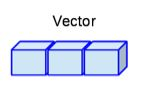Matrices

Two-dimensional array, rectangular data set of the same data type.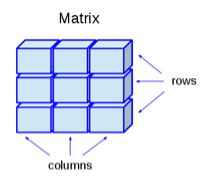Data Frames

Tabular data objects. Unlike a matrix. Each column in a data frame can contain different data type. The first column can be numeric while the second column can be character, and the thrid column can be logical. This can basically represent a database table, or an excel spread sheet.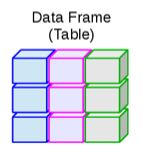Arrays

Arrays can be of any number of dimensions. Each dimension is a vector of the same type.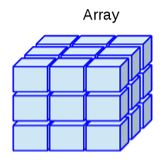Lists

Can contain many different types of elements inside it, like vectors, functions, and another list.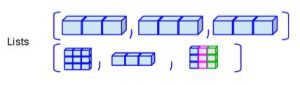Factors

Factors are R objects created using a vector. It automatically stores additional data like distinct valued of the elements in a vector as labels. Very useful in statistical modeling.

Sample Codes

Vectors

```> num_vector <- c(1,4,6,2.3)
> char_vector <- c("a", "b", "cd")
>
> num_vector
 1.0 4.0 6.0 2.3
> char_vector
 "a" "b" "cd"```

Matrices

```> M1 = matrix(c("a", "b", "b", "c", "d", "a"), nrow=3, ncol=3)
> M1
[,1] [,2] [,3]
[1,] "a" "c" "a"
[2,] "b" "d" "b"
[3,] "b" "a" "b"```

Data Frames

```> v1 = 1:4
> v2 = c("one","two","three","four")
> v3 <- c(T,F,T,F)
> df <- data.frame(v1,v2,v3)
> df
v1 v2 v3
1 1 one TRUE
2 2 two FALSE
3 3 three TRUE
4 4 four FALSE```

Arrays

```> a1 <- array(1:27, dim=c(3,3,3))
> a1
, , 1

[,1] [,2] [,3]
[1,] 1 4 7
[2,] 2 5 8
[3,] 3 6 9

, , 2

[,1] [,2] [,3]
[1,] 10 13 16
[2,] 11 14 17
[3,] 12 15 18

, , 3

[,1] [,2] [,3]
[1,] 19 22 25
[2,] 20 23 26
[3,] 21 24 27```

Lists

```> l1 <- list(c(1:4), TRUE, sin)
> l1
[]
 1 2 3 4

[]
 TRUE

[]
function (x) .Primitive("sin")```

Factors

```> colors <- c("red", "blue", "red", "green", "red", "blue")
> colors_factor <- factor(colors)
> colors_factor
 red blue red green red blue
Levels: blue green red```